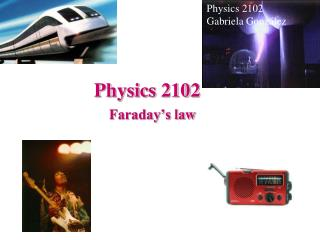DownloadDownload PresentationPhysics 2102

# Physics 2102

Download Presentation## Physics 2102

- - - - - - - - - - - - - - - - - - - - - - - - - - - E N D - - - - - - - - - - - - - - - - - - - - - - - - - - -
##### Presentation Transcript

1. Physics 2102 Gabriela González Physics 2102 Faraday’s law

2. n dA Faraday’s Law B A magnetic field can create a en electrical current too! If we define magnetic flux, similar to definition of electric flux, but for an open surface with an edge: Then atime varying magnetic FLUX creates an induced EMF, and thus an electrical current if the edge is a wire!: • Take note of the MINUS sign!! • The induced EMF acts in such a way that it OPPOSES the change in magnetic flux (“Lenz’s Law”).

3. Example • When the N pole approaches the loop, the flux “into” the loop (“downwards”) increases • The loop can “oppose” this change if a current were to flow clockwise, hence creating a magnetic flux “upwards.” • So, the induced EMF is in a direction that makes a current flow clockwise. • If the N pole moves AWAY, the flux “downwards” DECREASES, so the loop has a counter clockwise current!

4. Faraday’s law: Eddy Currents • A non-magnetic (e.g. copper, aluminum) ring is placed near a solenoid. • What happens if: • There is a steady current in the solenoid? • The current in the solenoid is suddenly changed? • The ring has a “cut” in it? • The ring is extremely cold?

5. Another Experimental Observation • Drop a non-magnetic pendulum (copper or aluminum) through an inhomogeneous magnetic field • What do you observe? Why? (Think about energy conservation!) Pendulum had kinetic energy What happened to it? Isn’t energy conserved?? N S

6. spark 12V http://www.familycar.com/Classroom/ignition.htm Example : the ignition coil • The gap between the spark plug in a combustion engine needs an electric field of ~107 V/m in order to ignite the air-fuel mixture. For a typical spark plug gap, one needs to generate a potential difference > 104 V! • But, the typical EMF of a car battery is 12 V. So, how does a spark plug work?? • Breaking the circuit changes the current through “primary coil” • Result: LARGE change in flux thru secondary -- large induced EMF! • The “ignition coil” is a double layer solenoid: • Primary: small number of turns -- 12 V • Secondary: MANY turns -- spark plug

7. n dA Another formulation of Faraday’s Law B • We saw that a time varying magnetic FLUX creates an induced EMF in a wire, exhibited as a current. • Recall that a current flows in a conductor because of electric field. • Hence, a time varying magnetic flux must induce an ELECTRIC FIELD! • Closed electric field lines!!?? No potential!! Another of Maxwell’s equations! To decide SIGN of flux, use right hand rule: curl fingers around loop, +flux -- thumb.

8. Path I: Path II: Example R2 R1 • The figure shows two circular regions R1 & R2 with radii r1 = 1m & r2 = 2m. In R1, the magnetic field B1 points out of the page. In R2, the magnetic field B2 points into the page. • Both fields are uniform and are DECREASING at the SAME steady rate = 1 T/s. • Calculate the “Faraday” integral for the two paths shown. I II

9. magnetic field lines electric field lines Example R • A long solenoid has a circular cross-section of radius R. • The current through the solenoid is increasing at a steady rate di/dt. • Compute the variation of the electric field as a function of the distance r from the axis of the solenoid. First, let’s look at r < R: Next, let’s look at r > R:

10. E(r) r r = R Example (continued)

11. Summary Two versions of Faradays’ law: • A varying magnetic flux produces an EMF: • A varying magnetic flux produces an electric field: